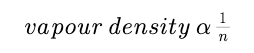# Degree of Dissociation (alpha) - Dependence of Degree of Dissociation on Density Measurements -Chemical Equilibrium

Degree of Dissociation (alpha)

let us consider the reaction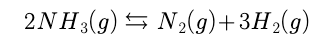Let the initial moles of NH3(g) be ‘a’. Let x moles of NH3 dissociate at equilibrium.initial moles             a                          0                  0

at Equilibrium          a -x                       x/2               3x/2

Degree of dissociation (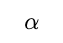) of NH3 is defined as the number of moles of NH3 dissociated per mole of NH3.

if x moles dissociate from ‘a’ moles of NH3, then, the degree of dissociation of NH3 would be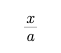We can also look at the reaction in the following manner.initial moles                        a                          0                  0

at Equilibrium                    a(1-alpha)                a*alpha/2               3a(alpha)/2

0r                                     a -2x'                          x'                            3x'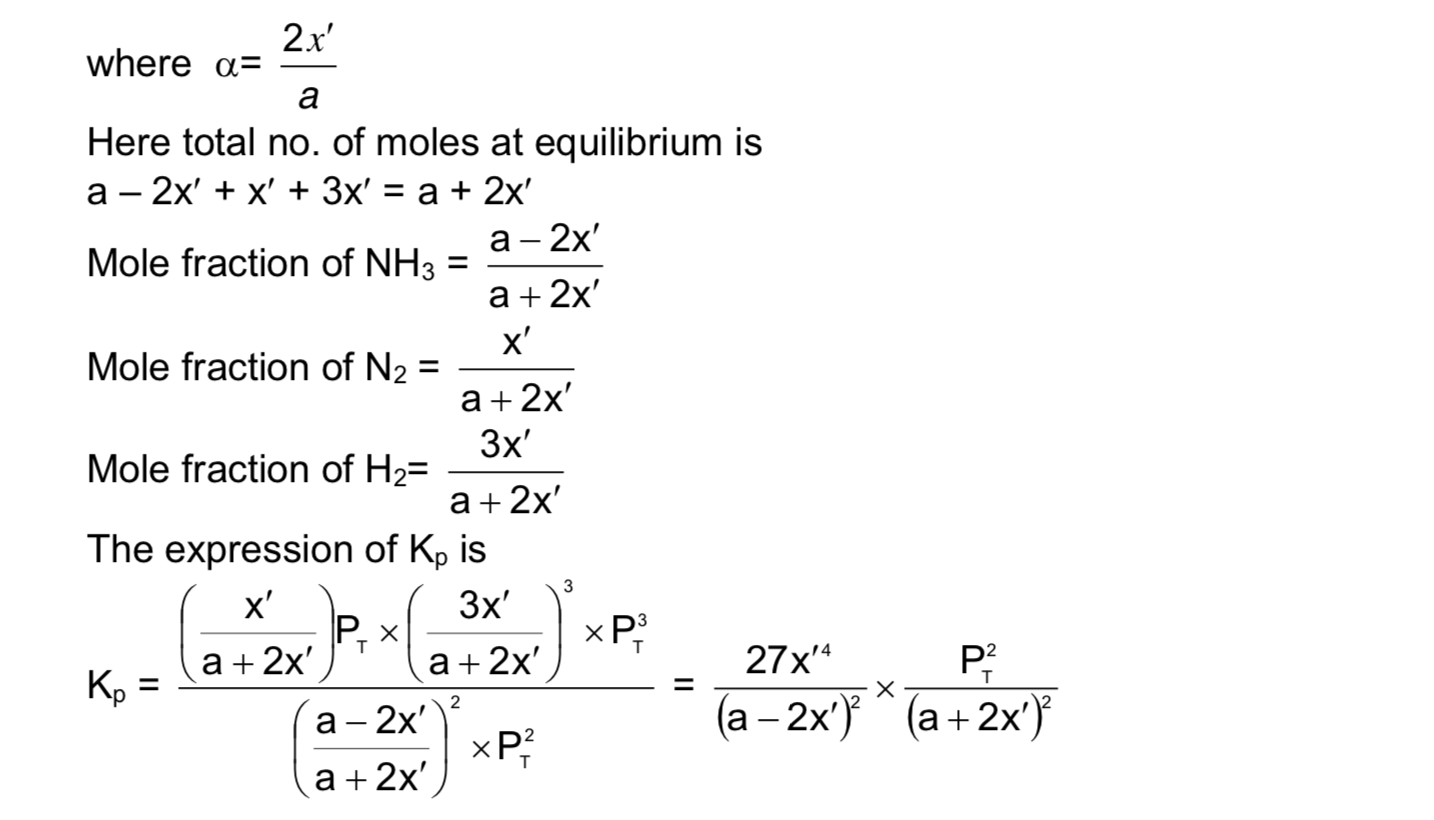Dependence of Degree of Dissociation on Density Measurements

The following is the method of calculating the degree of dissociation of a gas using vapour densities. This method is valid only for reactions whose KP exist, i.e., reactions having at least one gas and having no solution.

Since

PV = nRT

PV = (w/M) RT

M = wRT / PV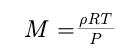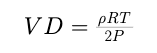since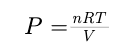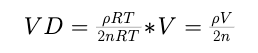For a reaction at eqb., V is a constant and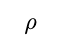is a constant.  i.e